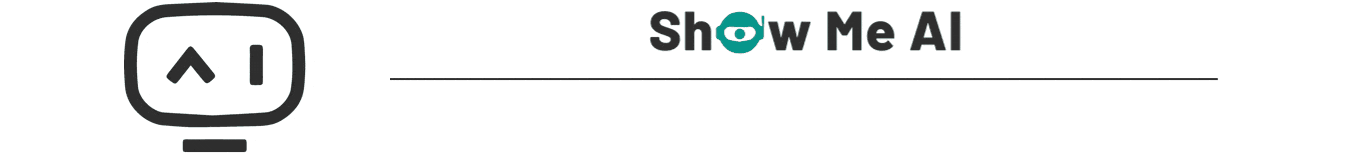# 深度学习与CV教程(7) | 神经网络训练技巧 (下)

2022-06-01 00:22 851 查看## 引言

ShowMeAI在上一篇 深度学习与CV教程(6) | 神经网络训练技巧 (上) 介绍了激活函数选择，sigmoid 和 tanh 都有饱和的问题；权重初始化不能太小也不能太大，最好使用Xavier初始化；数据预处理使用减去均值和归一化，线性分类中这两个操作会使分界线不那么敏感，即使稍微转动也可以，神经网络中也对权重的轻微改变没那么敏感，易于优化；也可以使用批量归一化，将输入数据变成单位高斯分布，或者缩放平移；学习过程跟踪损失、准确率；超参数体调优范围由粗到细，迭代次数逐渐增加，使用随机搜索。

• 更好的优化
• 正则化
• 迁移学习
• 模型集成

## 1.更好的优化（参数更新）

### 1.1 批梯度下降（BGD）

learning_rate

# 普通梯度下降
while True:
weights += -learning_rate * weights_grad  # 参数更新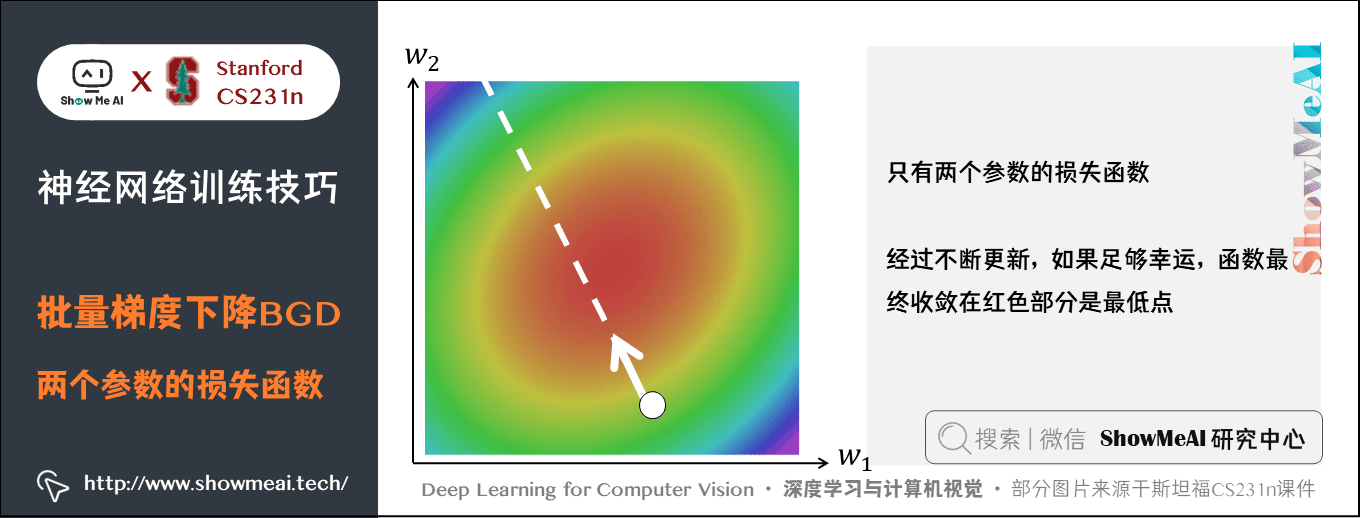• 优点：由于每一步都利用了训练集中的所有数据，因此当损失函数达到最小值以后，能够保证此时计算出的梯度为 0，能够收敛。因此，使用BGD时不需要逐渐减小学习率。
• 缺点：随着数据集的增大，运行速度会越来越慢。

### 1.2 随机梯度下降（SGD）

SGD的优点是训练速度快，对于很大的数据集，也能够以较快的速度收敛。但是实际应用SGD会有很多问题：

① 如果损失函数在一个参数方向下降的快另一个方向下降的慢，这样会导致 「 之字形 」下降到最低点，高维中很普遍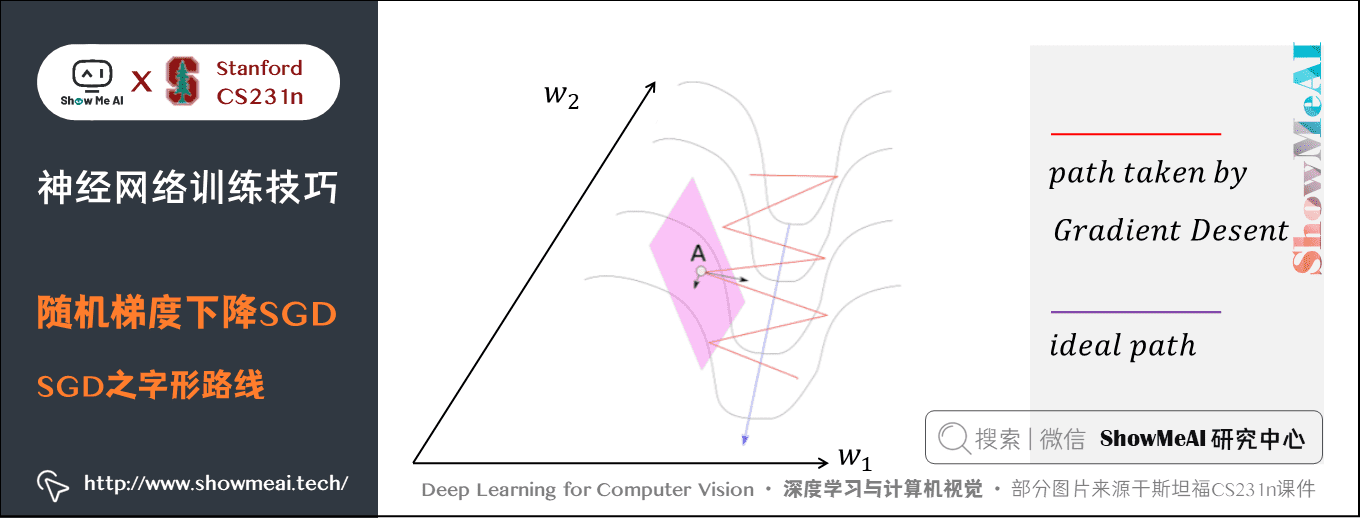• 上图是一个山沟状的区域，损失最小点沿着蓝色的线方向。考虑表面上的一个点A梯度，该点的梯度可以分解为两个分量，一个沿着方向 w_1，另一个沿着 w_2。
• 梯度在 w_1 方向上的分量要大得多，因为在 w_1 方向上每走一步都比在 w_2 方向损失值下降的多，虽然最小值在 w_2 方向上。这样实际走一步在 w_1 方向走的多， w_2 走得少，就会导致在这个沟里反复震荡，「之字形」前往最小值点。

② 如果损失函数有局部极小值和鞍点（既不是极大值也不是极小值的临界点）时，此时的梯度为$0$，参数更新会卡住，或在极小值附近震荡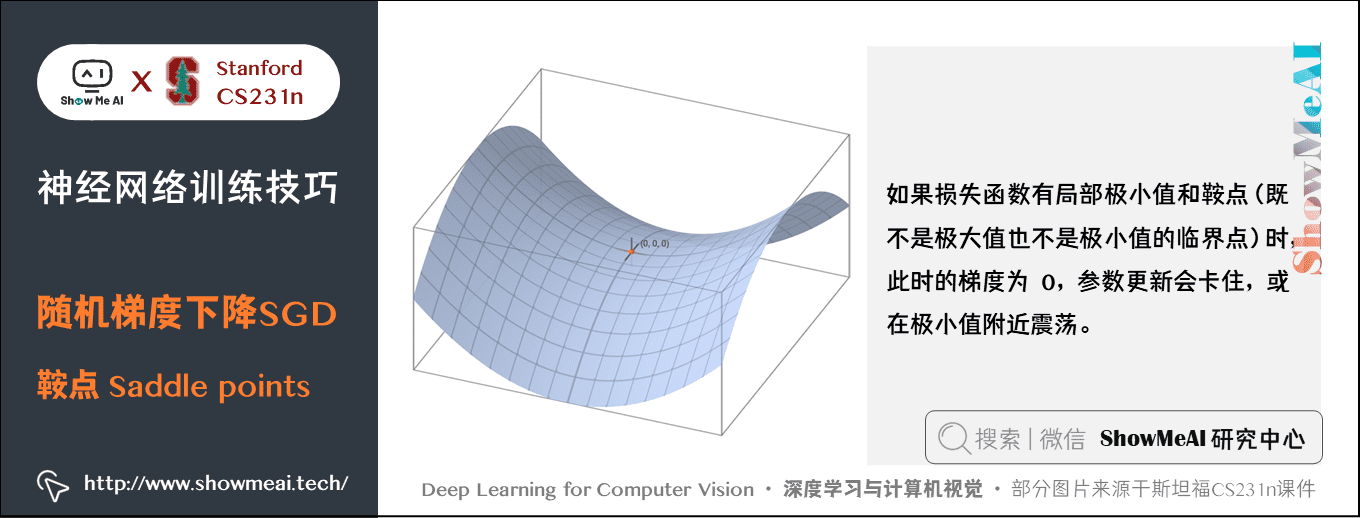• 在高维数据中，鞍点的存在是个更普遍也更大的问题，极小值每个梯度方向损失都会变大，而鞍点有的方向变大，有的减小，接近鞍点时更新缓慢。

③ SGD具有随机性，我们的梯度来自小批量数据（使用全部数据计算真实梯度速度太慢了），可能会有噪声，这样梯度下降的路线会很曲折，收敛的慢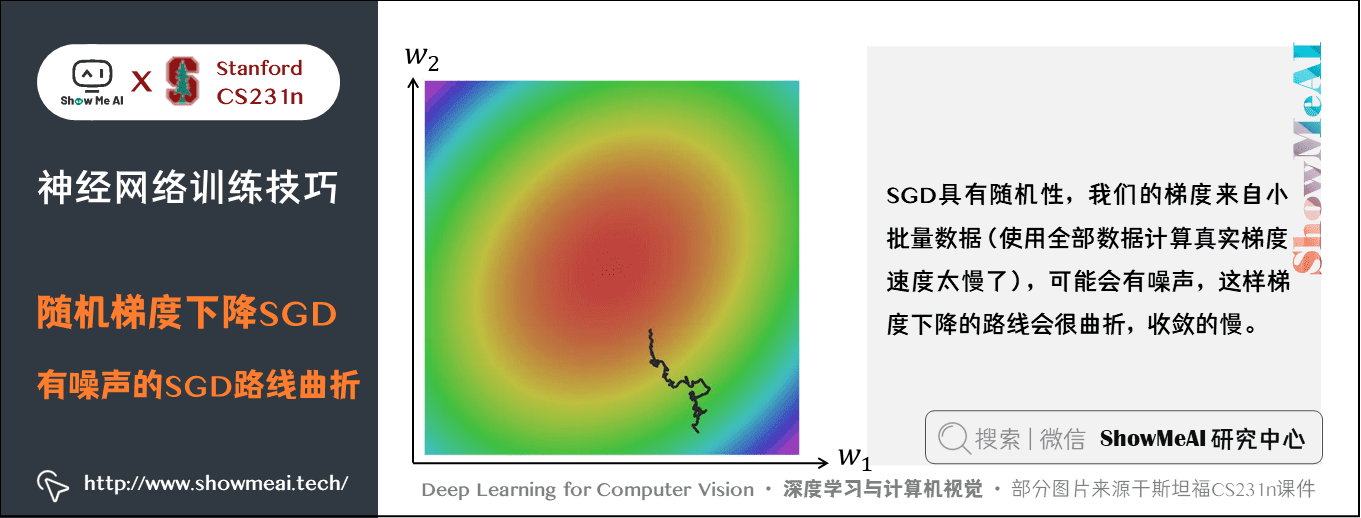### 1.3 动量（Momentum）更新

v=0
while True:
dW =  compute_gradient(W, X_train, y_train)
v = rho * v - learning_rate * dW
W += v
• rho
表示每回合速度
v
的衰减程度，每次迭代得到的梯度都是
dW
那么最后得到的
v
的稳定值为：\frac{-learning_{rate} \ast dw}{1-rho}
• rho
为 0 时表示 SGD，
rho
一般取值 0.5、0.9、0.99，对应学习速度提高两倍、10倍和100倍。

• 由于参数的更新要累积之前的梯度，所以如果我们分别累加这些梯度的两个分量，那么 w_1 方向上的分量将互相抵消，而 w_2 方向上的分量得到了加强。 但是由于衰减系数，不可能完全抵消，但是已经可以加速通过，很大程度缓解了「之字形」收敛慢的问题。这也是减少震荡的原理。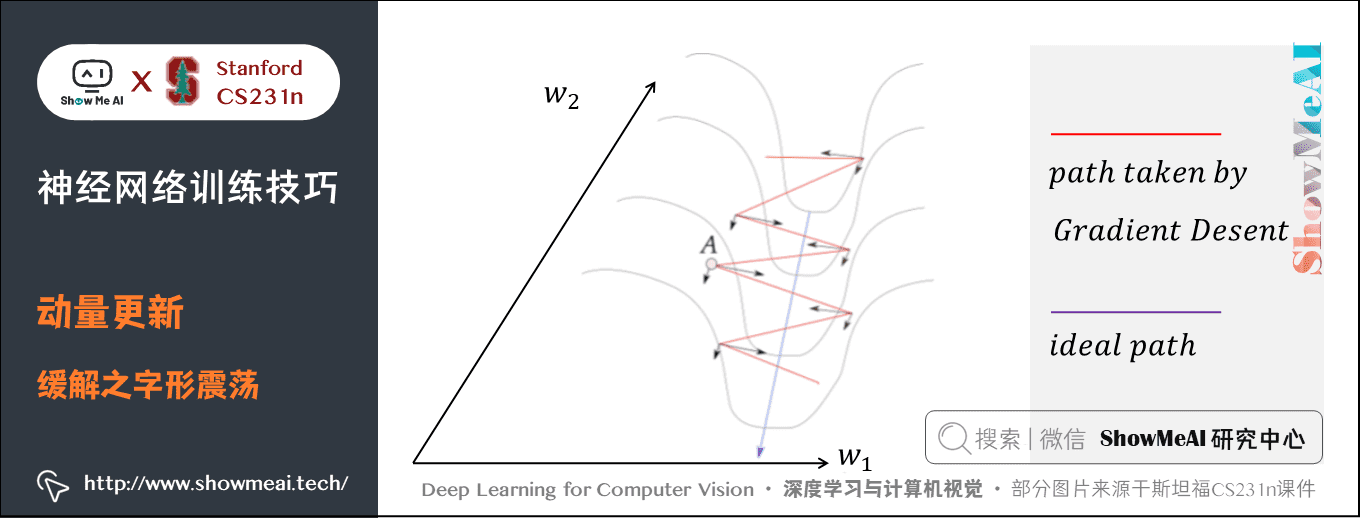• 局部最小值和鞍点由于还有之前的速度，会加速冲过去。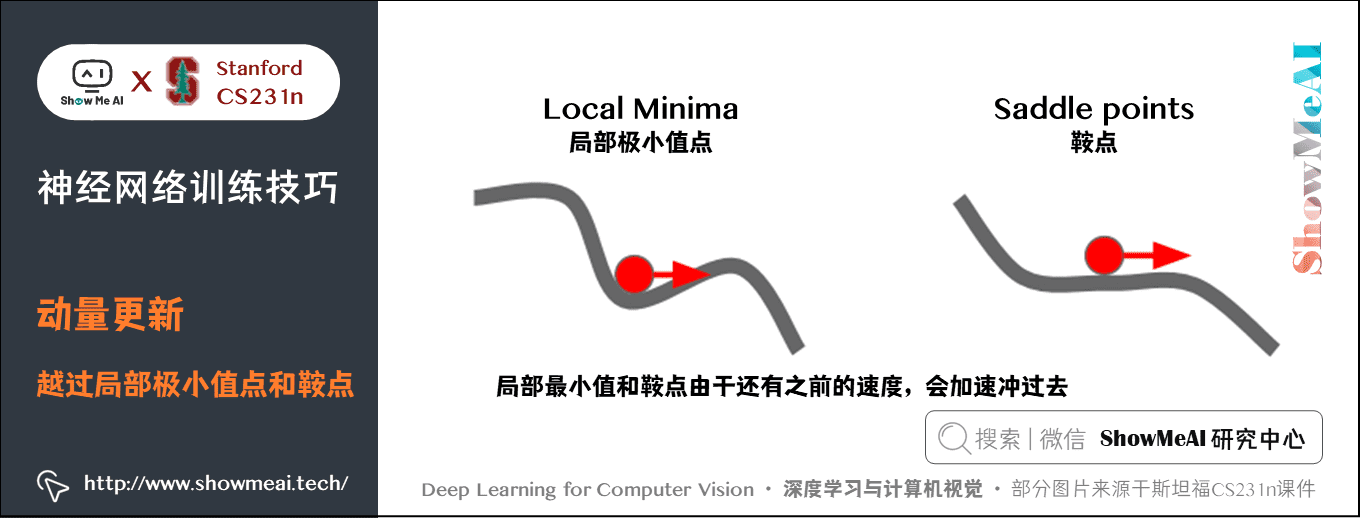• 面对梯度变化比较大的方向，即一些噪声，由于此时质点还有比较大的速度，这时的反方向需要先将速度减小为 0 才能改变参数更新方向，由于速度是累加的，所以个别的噪声的影响不会那么大，就可以平滑快速的收敛。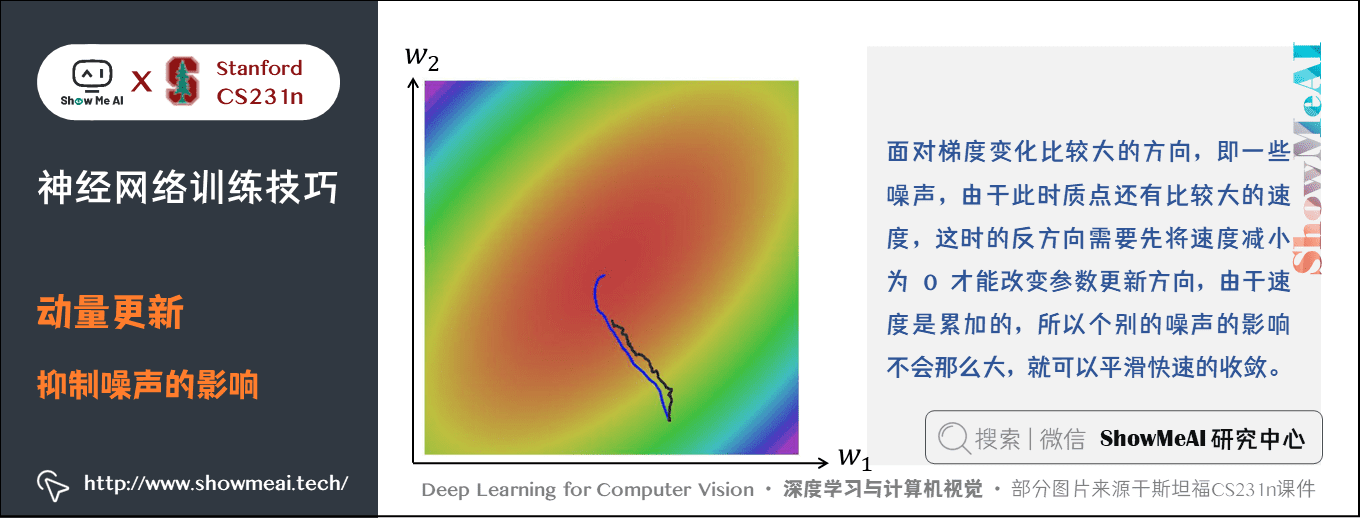### 1.4 Nesterov动量

Nesterov动量与普通动量有些许不同，最近变得比较流行。在理论上对于凸函数它能得到更好的收敛，在实践中也确实比标准动量表现更好一些。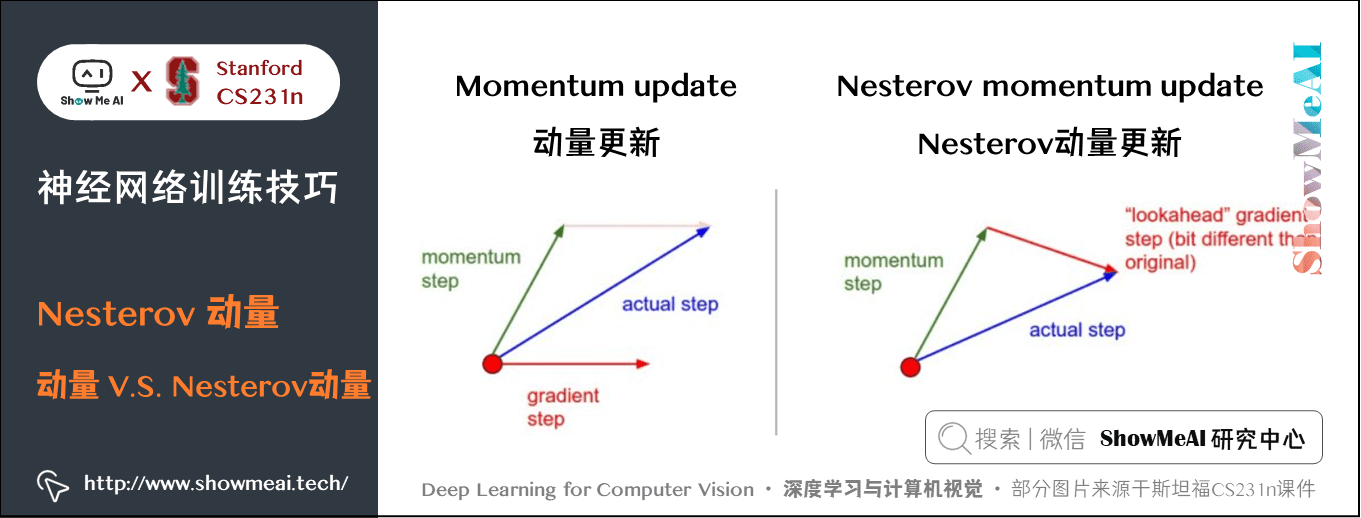• 普通的动量更新在某一点处有一个速度，然后计算该点的梯度，实际的更新方向会根据速度方向和梯度方向做一个权衡。
• Nesterov动量更新是既然我们知道动量将会将质点带到一个新的位置（即向前看），我们就不要在原来的位置计算梯度了，在这个「向前看」的地方计算梯度，更新参数。

v=0
while True:
W_ahead = W + rho * v
v = rho * v - learning_rate * dW_ahead
W += v

W_ahead = W + rho * v

W_ahead

W

v=0
while True:
pre_v = v
dW =  compute_gradient(W, X_train, y_train)
v = rho * v - learning_rate * dW
W += -rho * pre_v + (1 + rho) * v

v_{t+1}=\rho v_t - \alpha \nabla f(x_t+\rho v_t) x_{t+1}=x_t+v_{t+1}

v_{t+1}=\rho v_t-\alpha \nabla f(\tilde{x_t}) \begin{aligned} \tilde{x}_{t+1} &=x_{t+1}+\rho v_{t+1}\\ &=x_{t}+v_{t+1}+\rho v_{t+1}\\ &=\tilde{x}_{t}-\rho v_{t}+v_{t+1}+\rho v_{t+1} \end{aligned}

\tilde{x}_{t+1}=\tilde{x_t}-\rho v_t+(\rho+1)v_{t+1}
• 只更新 v_t 和 \tilde{x}_t 即可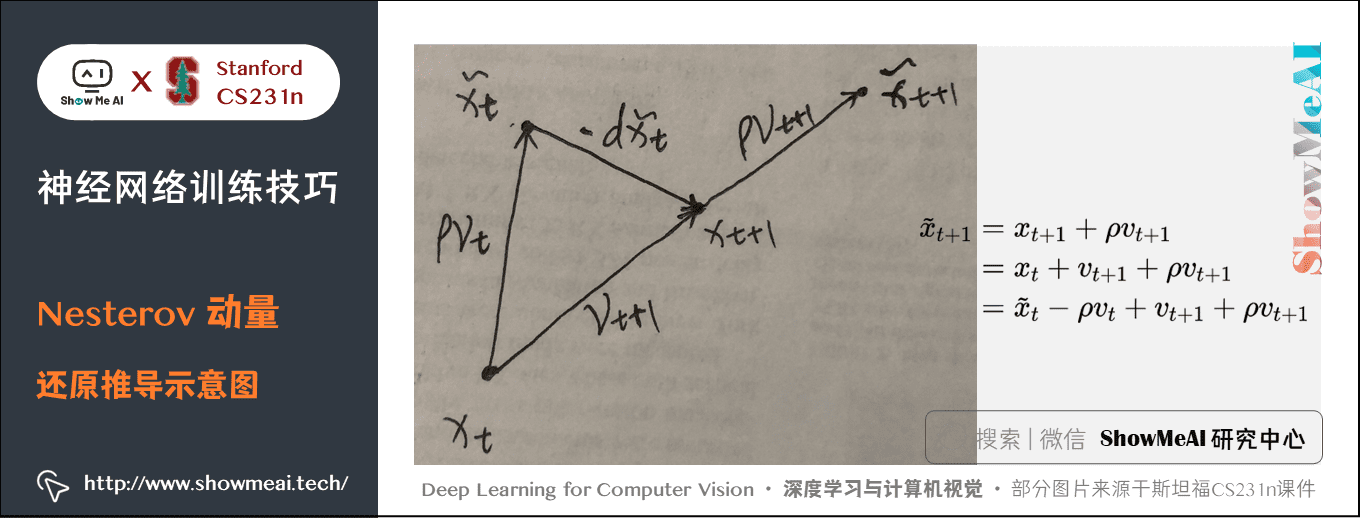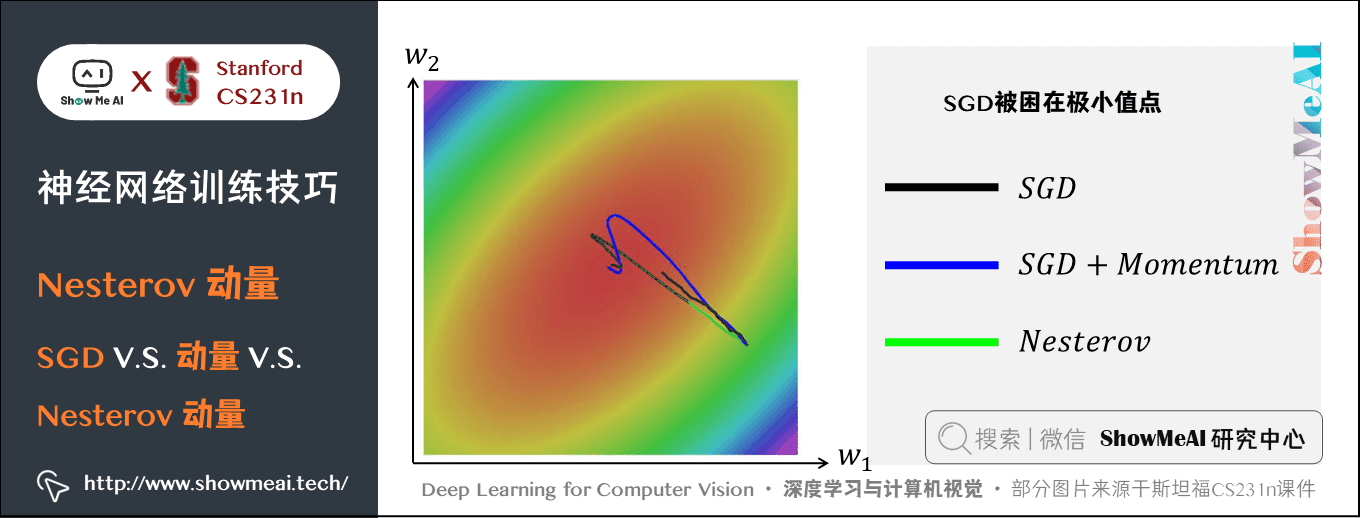eps = 1e-7
grad_squared =  $0$
while True:
grad_squared += dW * dW
W -= learning_rate * dW / (np.sqrt(grad_squared) + eps)

grad_squared

eps
（一般设为
1e-4
1e-8

• 优点：能够实现参数每一维的学习率的自动更改，如果某一维的梯度大，那么学习速率衰减的就快一些，延缓网络训练；如果某一维的梯度小，那么学习速率衰减的就慢一些，网络训练加快。
• 缺点：如果梯度累加的很大，学习率就会变得非常小，就会陷在局部极小值点或提前停（ RMSProp 算法可以很好的解决该问题）。

### 1.6 均方根支柱算法（RMSProp）

RMSProp优化算法也可以自动调整学习率，并且RMSProp为每个参数选定不同的学习率。

decay_rate

grad_squared

grad_squared =  decay_rate * grad_squared + (1 - decay_rate) * dx * dx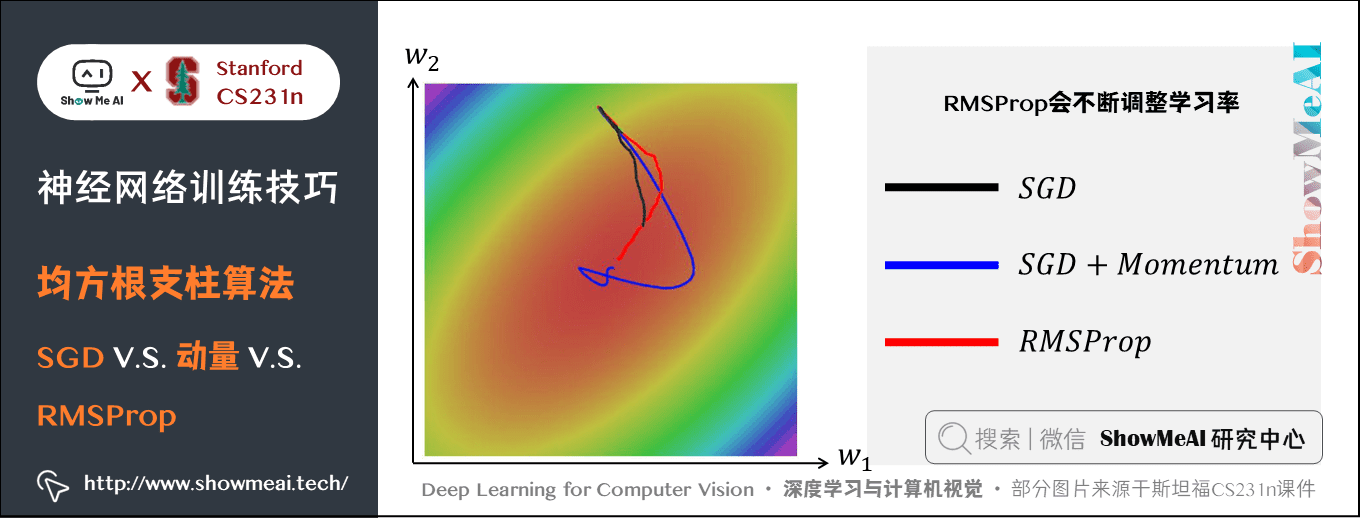eps = 1e-8
first_moment = 0  # 第一动量，用于累积梯度，加速训练
second_moment = 0  # 第二动量，用于累积梯度平方，自动调整学习率
while True:
first_moment = beta1 * first_moment + (1 - beta1) * dW  # Momentum
second_moment = beta2 * second_moment + (1 - beta2) * dW * dW  # AdaGrad / RMSProp
W -= learning_rate * first_moment / (np.sqrt(second_moment) + eps)

second_monent

eps = 1e-8
first_moment = 0  # 第一动量，用于累积梯度，加速训练
second_moment = 0  # 第二动量，用于累积梯度平方，自动调整学习率

for t in range(1, num_iterations+1):
first_moment = beta1 * first_moment + (1 - beta1) * dW  # Momentum
second_moment = beta2 * second_moment + (1 - beta2) * dW * dW  # AdaGrad / RMSProp
first_unbias = first_moment / (1 - beta1 ** t)  # 加入偏置，随次数减小，防止初始值过小
second_unbias = second_moment / (1 - beta2 ** t)
W -= learning_rate * first_unbias / (np.sqrt(second_unbias) + eps)

eps=1e-8
,
beta1=0.9
,
beta2=0.999
,
learning_rate = 1e-3
5e-4
，对大多数模型效果都不错。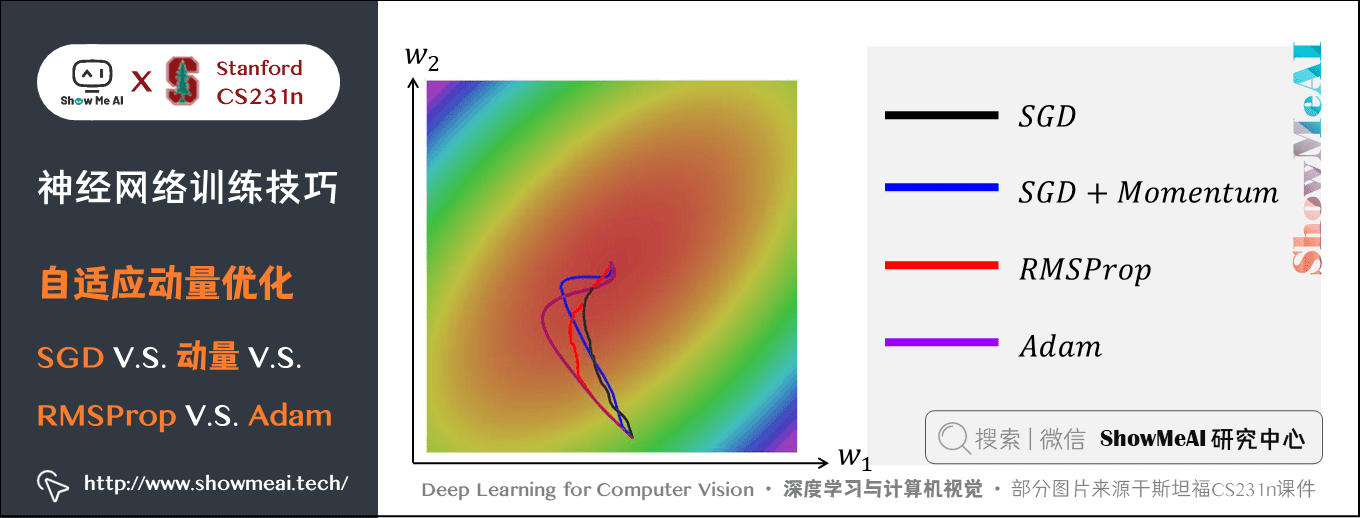### 1.8 学习率退火

• 如果学习率很高，系统的动能就过大，参数向量就会无规律地跳动，不能够稳定到损失函数更深更窄的部分去。
• 知道什么时候开始衰减学习率是有技巧的：慢慢减小它，可能在很长时间内只能是浪费计算资源地看着它混沌地跳动，实际进展很少。但如果快速地减少它，系统可能过快地失去能量，不能到达原本可以到达的最好位置。

① 随步数衰减：每进行几个周期（epoch）就根据一些因素降低学习率。典型的值是每过 5 个周期就将学习率减少一半，或者每 20 个周期减少到之前的 10%。

② 指数衰减：数学公式是 \alpha=\alpha_0e^{-kt}，其中 \alpha_0,k 是超参数， t 是迭代次数（也可以使用周期作为单位）。 ③ 1/t 衰减：数学公式是 \alpha=\alpha_0/(1+kt))，其中 \alpha_0,k 是超参数， t 是迭代次数。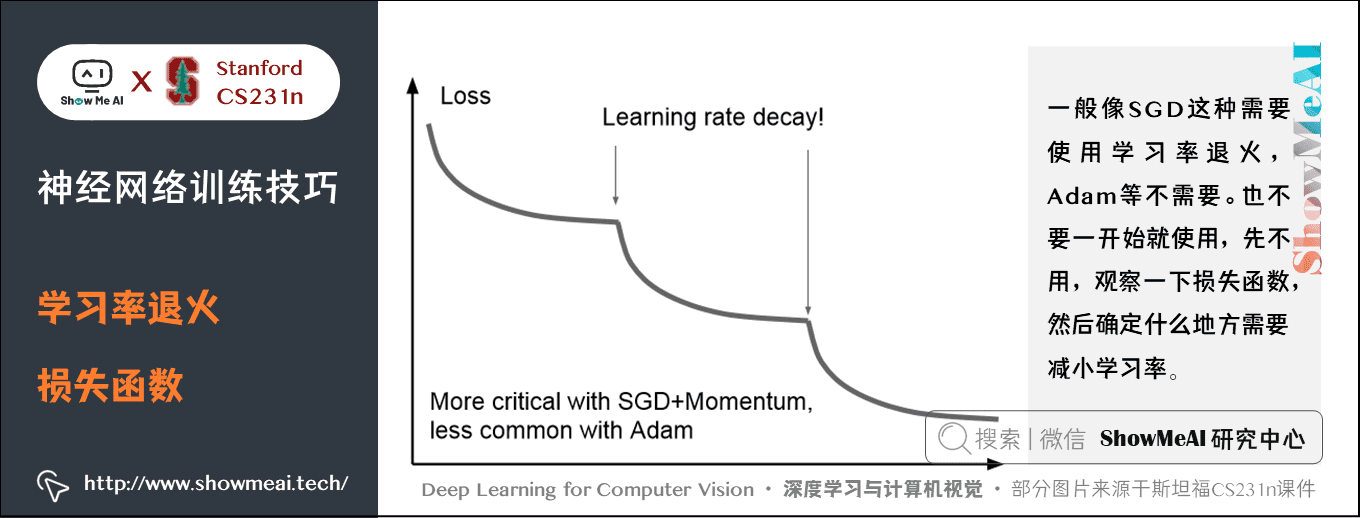### 1.9 二阶方法（Second-Order）

x \leftarrow x - [H f(x)]^{-1} \nabla f(x)

H f(x) 是 Hessian 矩阵，由 f(x) 的二阶偏导数组成：

\mathbf{H}=\left[\begin{array}{cccc} \frac{\partial^{2} f}{\partial x_{1}^{2}} & \frac{\partial^{2} f}{\partial x_{1} \partial x_{2}} & \cdots & \frac{\partial^{2} f}{\partial x_{1} \partial x_{n}} \\ \frac{\partial^{2} f}{\partial x_{2} \partial x_{1}} & \frac{\partial^{2} f}{\partial x_{2}^{2}} & \cdots & \frac{\partial^{2} f}{\partial x_{2} \partial x_{n}} \\ \vdots & \vdots & \ddots & \vdots \\ \frac{\partial^{2} f}{\partial x_{n} \partial x_{1}} & \frac{\partial^{2} f}{\partial x_{n} \partial x_{2}} & \cdots & \frac{\partial^{2} f}{\partial x_{n}^{2}} \end{array}\right]

x 是 n 维的向量，f(x) 是实数，所以海森矩阵是 n \ast n 的。

\nabla f(x) 是 n 维梯度向量，这和反向传播一样。

## 2.正则化

### 2.1 正则化的动机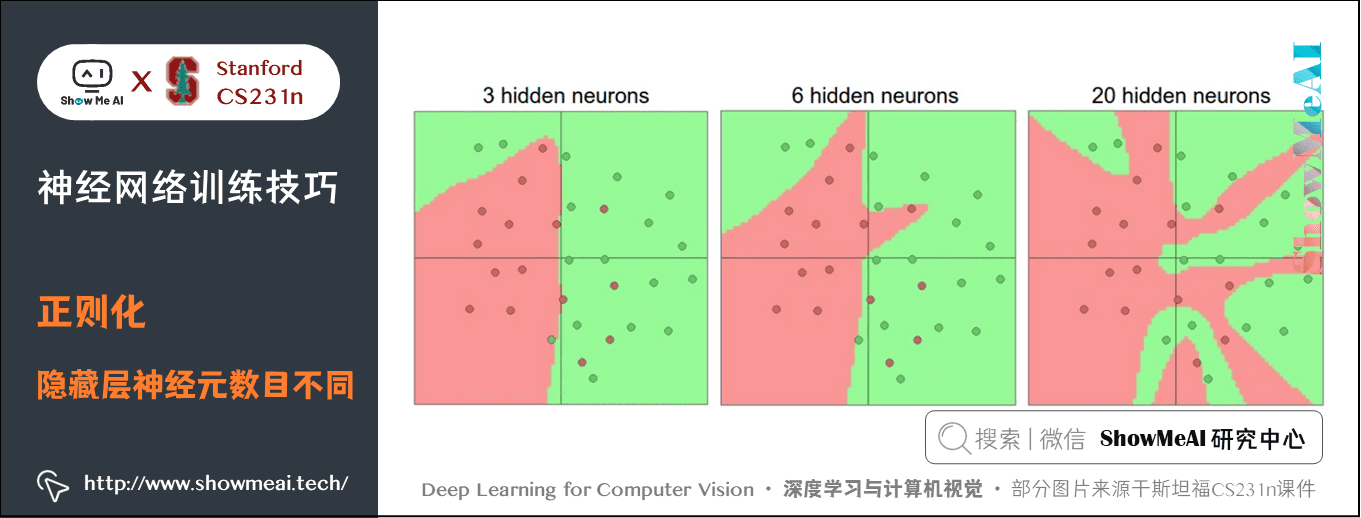• 优势是可以分类更复杂的数据
• 不足是可能造成对训练数据的过拟合。

• 有20个神经元隐层的网络拟合了所有的训练数据，但是其代价是把决策边界变成了许多不相连的红绿区域。
• 有3个神经元的模型的表达能力只能用比较宽泛的方式去分类数据。它将数据看做是两个大块，并把个别在绿色区域内的红色点看做噪声。在实际中，这样可以在测试数据中获得更好的泛化（generalization）能力。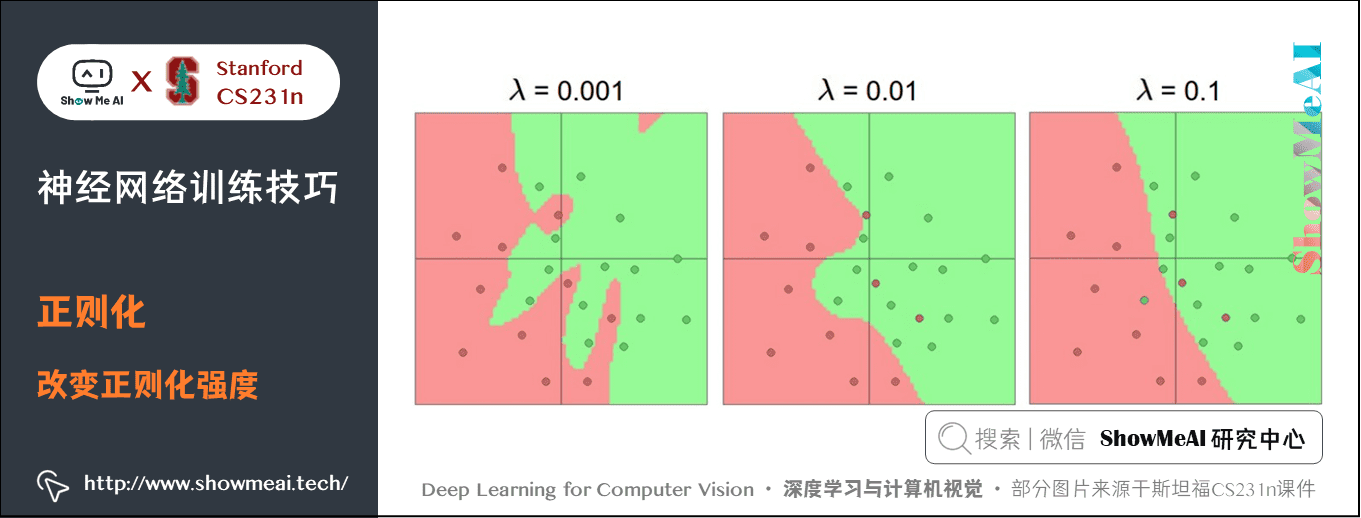ConvNetsJS demo 上有一个小例子大家可以练练手。

### 2.2 正则化方法

L2正则化：最常用的正则化，通过惩罚目标函数中所有参数的平方实现。

• 对于网络中的每个权重 w，向目标函数中增加一个 \frac{1}{2} \lambda w^2，1/2为了方便求导， \lambda 是正则强度。
• L2 正则化可以直观理解为它对于大数值的权重向量进行严厉惩罚，倾向于更加分散的权重向量。使网络更倾向于使用所有输入特征，而不是严重依赖输入特征中某些小部分特征。

L1正则化：是另一个相对常用的正则化方法，对于每个 w 都向目标函数增加一个 \lambda \mid w \mid。

• L1 正则化会让权重向量在最优化的过程中变得稀疏（即非常接近 0）。在实践中，如果不是特别关注某些明确的特征选择，一般说来 L2 正则化都会比 L1 正则化效果好。
• L1 和 L2 正则化也可以进行组合： \lambda_1 \mid w \mid + \lambda_2 w^2，称作 Elastic net regularization。

### 2.3 随机失活（Dropout）

#### 1) Dropout概述

Dropout 是一个简单又极其有效的正则化方法，由 Srivastava 在论文 [Dropout: A Simple Way to Prevent Neural Networks from Overfitting](http://www.cs.toronto.edu/~rsalakhu/papers/srivastava14a.pdf) 中提出，与 L1 正则化、L2 正则化和最大范式约束等方法互为补充。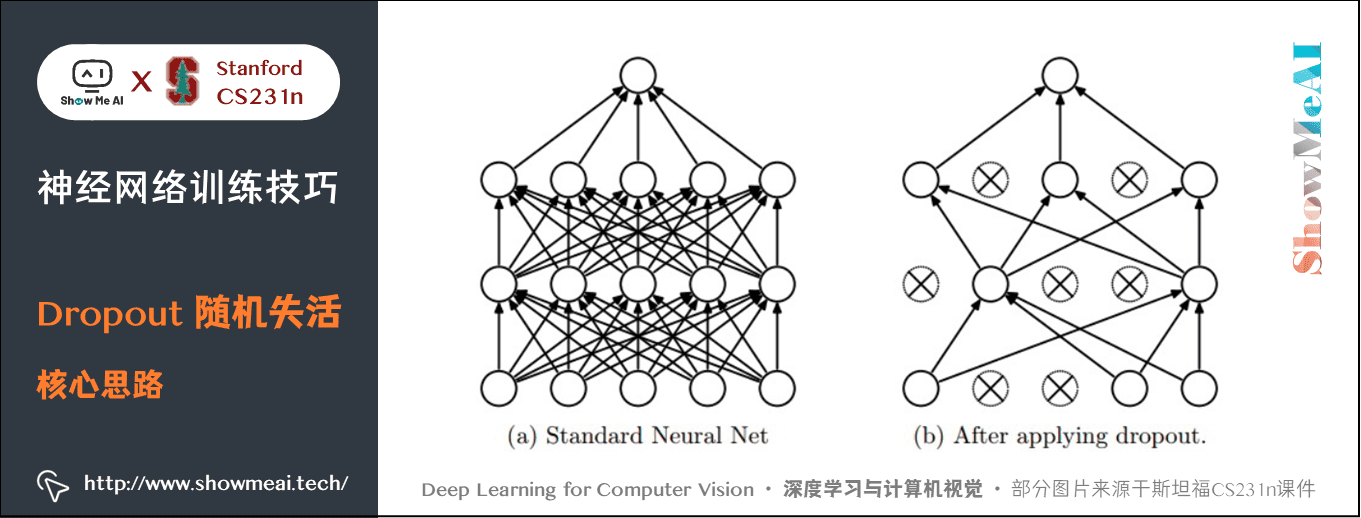""" 普通版随机失活"""

p = 0.5   # 神经元被激活的概率。p值越高，失活数目越少

def train_step(X):
""" X中是输入数据 """
# 前向传播
H1 = np.maximum(0, np.dot(W1, X) + b1)
U1 = np.random.rand(*H1.shape) < p # 第一个随机失活掩模
# rand可以返回一个或一组服从“0~1”均匀分布的随机样本值
# 矩阵中满足小于p的元素为True，不满足False
# rand()函数的参数是两个或一个整数，不是元组，所以需要*H1.shape获取行列
H1 *= U1 # U1中False的H1对应位置置零
H2 = np.maximum(0, np.dot(W2, H1) + b2)
U2 = np.random.rand(*H2.shape) < p # 第二个随机失活掩模
H2 *= U2 # drop!
out = np.dot(W3, H2) + b3

# 反向传播:计算梯度... (略)
# 进行参数更新... (略)

train_step

#### 2) Dropout的理解

• 比如每个神经元学到了猫的一个特征比如尾巴、胡须、爪子等，将这些特征全部组合起来可以判断是一只猫。
• 加入随机失活后就只能依赖一些零散的特征去判断不能使用所有特征，这样可以一定程度上抑制过拟合。不然训练时正确率很高，测试时却很低。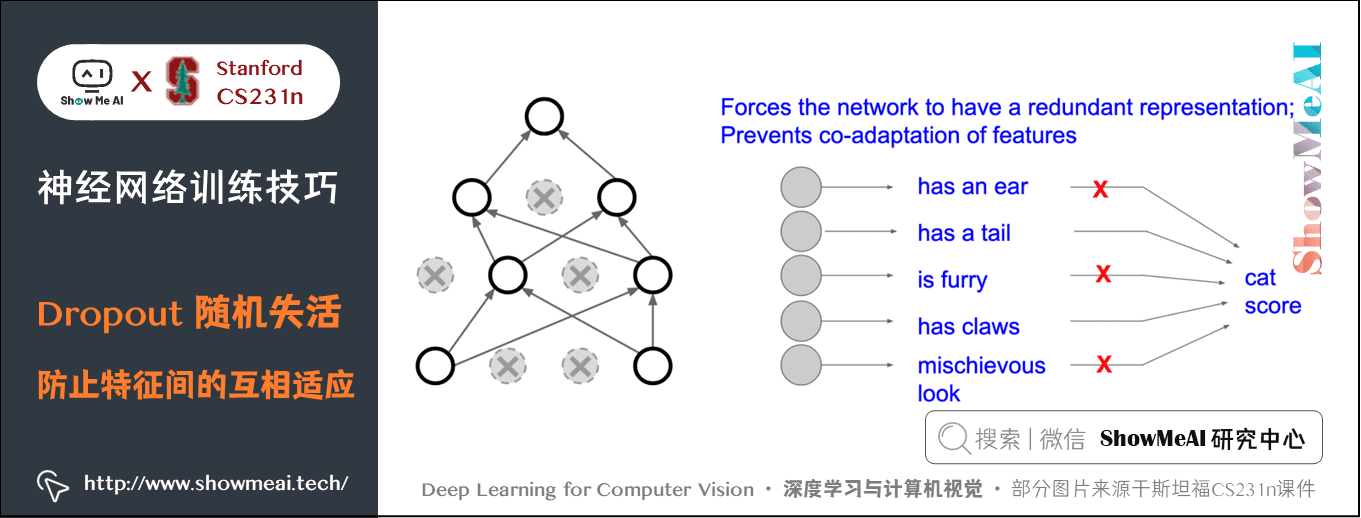• 在训练过程中，随机失活可以被认为是对完整的神经网络抽样出一些子集，每次基于输入数据只更新子网络的参数。
• 每个二值掩模都是一个模型，有 n 个神经元的网络有 2n 种掩模。Dropout 相当于数量巨大的网络模型（共享参数）在同时被训练。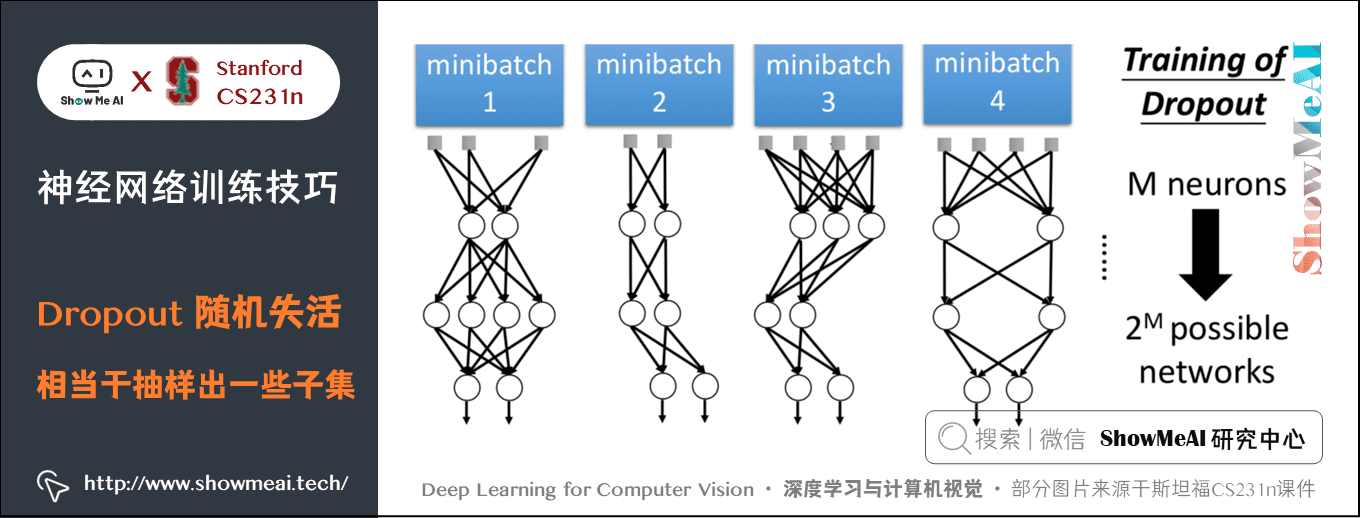#### 3) 测试时避免随机失活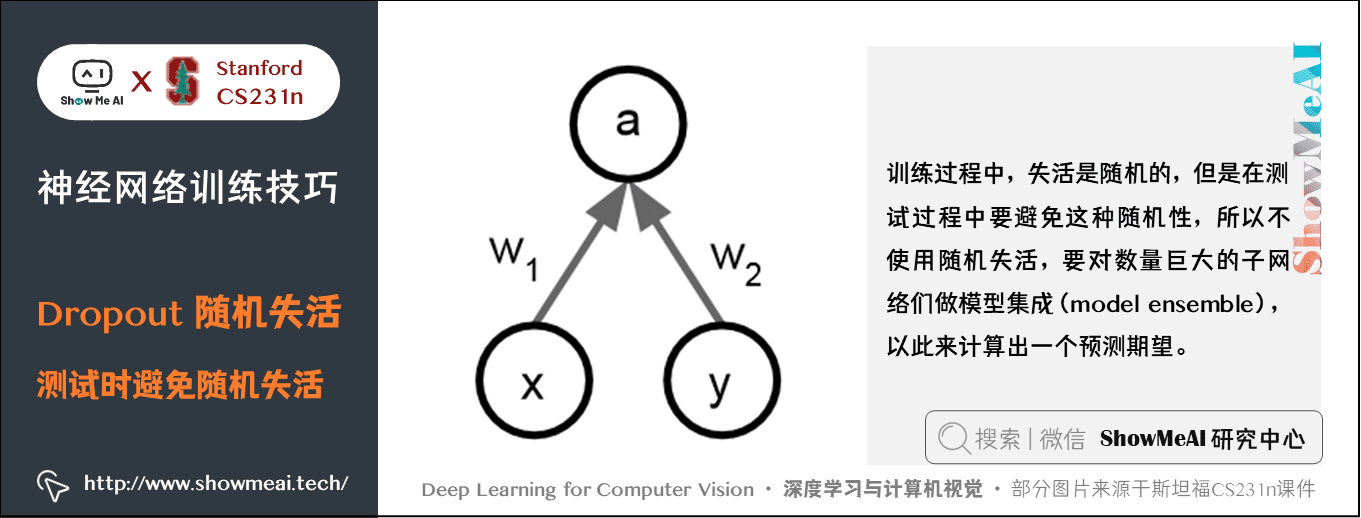\text{E}(a)=w_1x+w_2y

\text{E}(a)=\frac{1}{4}(w_1x+w_2y)+\frac{1}{2}(w_1x+w_2\cdot 0)+\frac{1}{2}(w_1x\cdot 0+w_2)+\frac{1}{4}\cdot 0=\frac{1}{2}(w_1x+w_2y)

def predict(X):
# 前向传播时模型集成
H1 = np.maximum(0, np.dot(W1, X) + b1) * p # 注意：激活数据要乘以p
H2 = np.maximum(0, np.dot(W2, H1) + b2) * p # 注意：激活数据要乘以p
out = np.dot(W3, H2) + b3

• 在训练时就进行数值范围调整，从而让前向传播在测试时保持不变。

"""

"""
p = 0.5
def train_step(X):
# 前向传播
H1 = np.maximum(0, np.dot(W1, X) + b1)
U1 = (np.random.rand(*H1.shape) < p) / p # 第一个随机失活遮罩. 注意/p!
H1 *= U1 # drop!
H2 = np.maximum(0, np.dot(W2, H1) + b2)
U2 = (np.random.rand(*H2.shape) < p) / p # 第二个随机失活遮罩. 注意/p!
H2 *= U2 # drop!
out = np.dot(W3, H2) + b3

def predict(X):
# 前向传播时模型集成
H1 = np.maximum(0, np.dot(W1, X) + b1) # 不用数值范围调整了
H2 = np.maximum(0, np.dot(W2, H1) + b2)
out = np.dot(W3, H2) + b3

• 批量归一化：训练时的均值和方差来自随机的小批量；测试时使用的是整个训练过程中的经验方差和均值。

• 数据增强（data augmentation） ：比如一张猫的图片进行训练时，可以随机的裁剪翻转等操作再训练，然后测试过程再对一些固定的位置（四个角、中心及翻转）进行测试。也可以在训练的时候随机改变亮度对比度，色彩抖动PCA降维等。

• DropConnect：另一个与 Dropout 类似的研究是 DropConnect，它在前向传播的时候，一系列权重被随机设置为 0 。

• 部分最大池化（Fractional Max Pooling） ：训练时随机区域池化，测试时固定区域或者取平均值。这个方法并不常用。

• 随机深度（Stochastic Depth） ：一个比较深的网络，训练时随机选取部分层去训练，测试时使用全部的层。这个研究非常前沿。

#### 4) 实践经验

• 可以通过交叉验证获得一个全局使用的 L2 正则化系数。
• 使用 L2 正则化的同时在所有层后面使用随机失活

## 3.迁移学习（Transfer Learning）

### 3.1 迁移学习的思想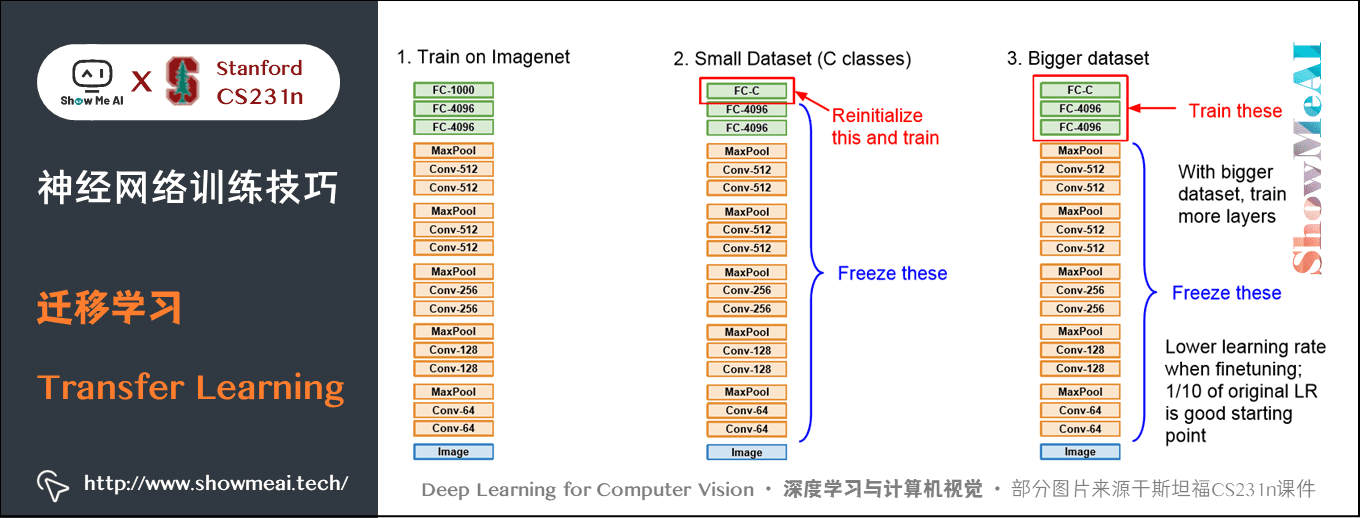• 第①步：在大量的数据集上训练一个 CNN，得到模型（比如使用 ImageNet，有 1000 个分类）
• 第②步：使用一个少量的数据集，最后需要的得到的分类也不再是 1000 而是一个较小的值 C，比如10。这时最后一个全连接层的参数矩阵变成 4096 \times C，初始化这个矩阵，重新训练这个线性分类器，保持前面的所有层不变，因为前面的层已经训练好了，有了泛化能力。
• 第③步：当得到较多的训练集后，训练的层数可以增多，比如可以训练最后三个全连接层。可以使用较低的学习率微调参数。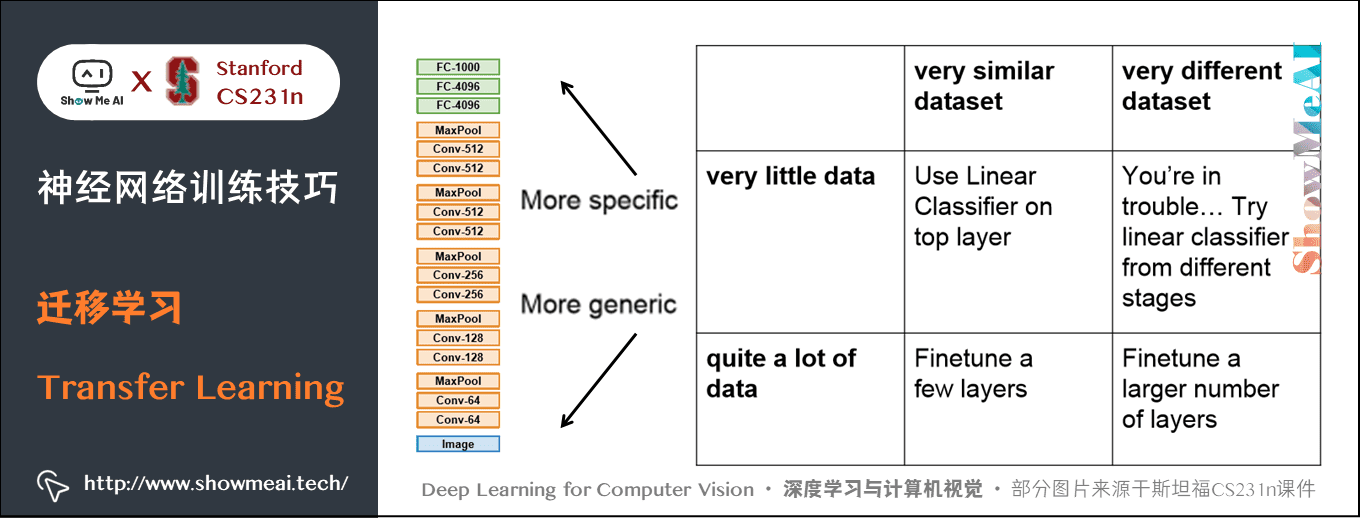## 4.模型集成（Model Ensembles）

• 同一个模型，不同的初始化。使用交叉验证来得到最好的超参数，然后用最好的参数来训练不同初始化条件的模型。这种方法的风险在于多样性只来自于不同的初始化条件。
• 在交叉验证中发现最好的模型。使用交叉验证来得到最好的超参数，然后取其中最好的几个（比如10个）模型来进行集成。这样就提高了集成的多样性，但风险在于可能会包含不够理想的模型。在实际操作中，这样操作起来比较简单，在交叉验证后就不需要额外的训练了。
• 一个模型设置多个记录点 （ checkpoints ） 。如果训练非常耗时，那就在不同的训练时间对网络留下记录点（比如每个周期结束），然后用它们来进行模型集成。很显然，这样做多样性不足，但是在实践中效果还是不错的，这种方法的优势是代价比较小。
• 在训练的时候跑参数的平均值。和上面一点相关的，还有一个也能得到1-2个百分点的提升的小代价方法，这个方法就是在训练过程中，如果损失值相较于前一次权重出现指数下降时，就在内存中对网络的权重进行一个备份。这样你就对前几次循环中的网络状态进行了平均。你会发现这个「平滑」过的版本的权重总是能得到更少的误差。直观的理解就是目标函数是一个碗状的，你的网络在这个周围跳跃，所以对它们平均一下，就更可能跳到中心去。

## 6.要点总结

• 正则化：L2比较常用，Dropout也是一个很好的正则方法。
• 数据较少时可以使用迁移学习。
• 模型集成。

## ShowMeAI 系列教程推荐